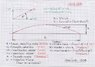# Projectile Motion Equations

• TimeInquirer

#### TimeInquirer

I was recently doing a few projectile motion equations and noticed that the formula used changes depending on whether or not a given initial position. Am I correct, or am I misinterpreting the situation and manipulation of the equation? Also, can someone provide a file or pdf for all the projectile motion equations? Thank you very much.

Hi TimeInquirer,

When you post a question here in the homework sections of Physics Forums we require that the formatting template be used regardless of the nature of the inquiry. Please keep this in mind for future posts. Your query could have been better formulated with specific examples to demonstrate your point.

If a given problem does not provide an initial position and an initial position is irrelevant to the nature of the given problem, then sure, the initial position parameter will be dropped if the required equation has one, or more likely, a different kinematic equation will be required for the given set of known values and the result sought (see in particular the SUVAT equations).

Regarding your request for a list of equations, note that there is a pinned thread at the top of the thread list titled "Introductory Physics Formulary". Take a look there.

Thank you. I understand what is needed now. However, I was not able to find a set of projectile motion equations in that thread.

Thank you. I understand what is needed now. However, I was not able to find a set of projectile motion equations in that thread.
The basic equations are covered under the heading Basic Equations of 1-D Kinematics. Projectile motion employs the same equations (the ones that include acceleration are used for the vertical component of the motion). Related formulas are those pertaining to Work, Mechanical Energy, and Power under the heading of the same name.

This attachement might help (it may not):

#### Attachments

•p008.jpg
82.8 KB · Views: 388
Dear TI,

One is that once you understand the matter at college level, you don't need a sheet with "all the equations": you know that it's linear uniform motion in one direction and linear motion with constant acceleration in the other direction, independent of and ##\perp## the first. And at your level you can whip up the integrations from a to v to x or y in no time.

Another is that you know exactly what to look for -- witness the title you chose for this thread. Google it and you can eat your heart out at all the stuff you can possibly think of.

But then I'm really puzzled you need to be pointed explicitly to the heading basic equations of 1D kinematics.

As I said, surprised, puzzled and somewhat intrigued,
BvU

Dear TI,

One is that once you understand the matter at college level, you don't need a sheet with "all the equations": you know that it's linear uniform motion in one direction and linear motion with constant acceleration in the other direction, independent of and ##\perp## the first. And at your level you can whip up the integrations from a to v to x or y in no time.

Another is that you know exactly what to look for -- witness the title you chose for this thread. Google it and you can eat your heart out at all the stuff you can possibly think of.

But then I'm really puzzled you need to be pointed explicitly to the heading basic equations of 1D kinematics.

As I said, surprised, puzzled and somewhat intrigued,
BvU
No need to mention your reaction 3 times in one post. It was just a way to make sure the all the necessary equations were in place and to avoid spending an hour looking at high school level equations (I was hoping there would be a pdf file someone had already shared) .

"But then I'm really puzzled you need to be pointed explicitly to the heading basic equations of 1D kinematics"- I am really puzzled that you were unable to see the starter banner on my icon...